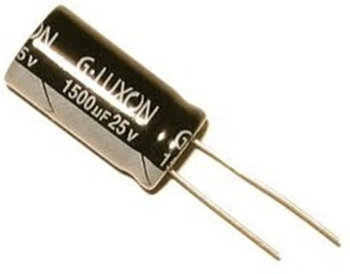﻿ How Long Does It Take to Charge a Capacitor?# How Long Does It Take to Charge a Capacitor?A capacitor charges to 63% of the supply voltage that is charging it after one time period. After 5 time periods, a capacitor charges up to over 99% of its supply voltage. Therefore, it is safe to say that the time it takes for a capacitor to charge up to the supply voltage is 5 time constants.

To calculate the time constant of a capacitor, the formula is τ=RC. This value yields the time (in seconds) that it takes a capacitor to charge to 63% of the voltage that is charging it up. After 5 time constants, the capacitor will charged to over 99% of the voltage that is supplying.

Therefore, the formula to calculate how long it takes a capacitor to charge to is:

Time for a Capacitor to Charge= 5RC

After 5 time constants, for all extensive purposes, the capacitor will be charged up to very close to the supply voltage. A capacitor never charges fully to the maximum voltage of its supply voltage, but it gets very close.

### Example

Below we have a circuit of a 9-volt battery charging a 1000µF capacitor through a 3KΩ resistor:One time constant, τ=RC=(3KΩ)(1000µF)=3 seconds. 5x3=15 seconds. So it takes the capacitor about 15 seconds to charge up to near 9 volts.